# 3. Redox Potentials for Other Systems

p{
color: blue;
font-family: Helvetica, Arial
}

Measuring redox potentials for more complicated systems

Systems involving gases

The obvious example here is chlorine. Chlorine is well known as an oxidizing agent. Since the electrochemical series is about ranking substances according to their oxidizing or reducing ability, it makes sense to include things like chlorine.

This time we are measuring the position of this equilibrium relative to the hydrogen equilibrium.Notice that the equilibrium is still written with the electrons on the left-hand side of the equation. That's why the chlorine gas has to appear on the left-hand side rather than on the right (which is where the metals and hydrogen appeared).

How can this equilibrium be connected into a circuit? The half-cell is built just the same as a hydrogen electrode. Chlorine gas is bubbled over a platinum electrode, which is immersed in a solution containing chloride ions with a concentration of 1 mol dm-3.

The conventional way of writing the whole cell looks like this.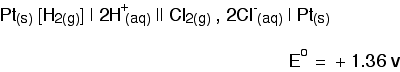Notice the way that the chlorine half cell is written. The convention is that the substance losing electrons is written closest to the electrode. In this case, the chloride ions are losing electrons.

If you had the chlorine half cell on the left-hand side in a different situation, then the convention still has to hold. The half cell would then be written: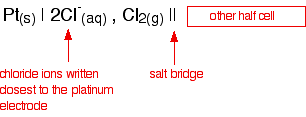What does the E° value show in the Cl2 / Cl - case?

The value is positive and moderately high as E° values go. That means that the position of the Cl2 / Cl - equilibrium lies more to the right than the hydrogen equilibrium. Chlorine is much more likely to pick up electrons than hydrogen ions are.Chlorine is therefore quite good a removing electrons from other things. It is a good oxidizing agent.

Measuring redox potentials for other systems

The Fe2+ / Fe3+ system

Iron(II) ions are easily oxidized to iron(III) ions, and iron(III) ions are fairly easily reduced to iron(II) ions. The equilibrium we are interested in this time is: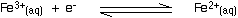To measure the redox potential of this, you would simply insert a platinum electrode into a beaker containing a solution containing both iron(II) and iron(III) ions (1 mol dm-3 with respect to both), and couple this to a hydrogen electrode.

The cell diagram would look like this:Notice that the E° value isn't as positive as the chlorine one. The position of the iron(III) / iron(II) equilibrium isn't as far to the right as the chlorine equilibrium. That means that Fe3+ ions don't pick up electrons as easily as chlorine does. Chlorine is a stronger oxidizing agent than Fe3+ ions.

Potassium dichromate(VI) as an oxidizing agent

A commonly used oxidizing agent, especially in organic chemistry, is potassium dichromate(VI) solution acidified with dilute sulfuric acid. The potassium ions are just spectator ions and aren't involved in the equilibrium in any way.

The equilibrium is more complicated this time because it contains more things:The half cell would have a piece of platinum dipping into a solution containing all the ions (dichromate(VI) ions, hydrogen ions and chromium(III) ions) all at 1 mol dm-3.

There is yet another convention when it comes to writing these more complicated cell diagrams. Where there is more than one thing on either side of the equilibrium, square brackets are written around them to keep them tidy. The substances losing electrons are written next to the electrode, just as before.Including these new redox potentials in the electrochemical series

These values can be slotted seamlessly into the electrochemical series that so far has only included metals and hydrogen.

An updated electrochemical series

equilibrium E° (volts)-3.03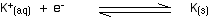-2.92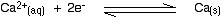-2.87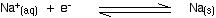-2.71-2.37-1.66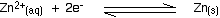-0.76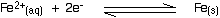-0.44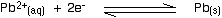-0.13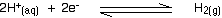0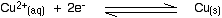+0.34+0.77+0.80+1.33+1.36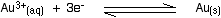+1.50

By coincidence, all the new equilibria we've looked at have positive E° values. It so happens that most of the equilibria with negative E° values that you meet at this level are ones involving simple metal / metal ion combinations.

An update on oxidising agents

Remember:

• The more positive the E° value, the further the position of equilibrium lies to the right.

• That means that the more positive the E° value, the more likely the substances on the left-hand side of the equations are to pick up electrons.

• A substance which picks up electrons from something else is an oxidizing agent.

• The more positive the E° value, the stronger the substances on the left-hand side of the equation are as oxidizing agents.

Of the new ones we've added to the electrochemical series:

• Chlorine gas is the strongest oxidizing agent (E° = +1.36 v).

• A solution containing dichromate(VI) ions in acid is almost as strong an oxidizing agent (E° = +1.33 v).

• Iron(III) ions are the weakest of the three new ones (E° = +0.77 v).

• None of these three are as strong an oxidizing agent as Au3+ ions (E° = +1.50 v).

The Rubik's Cube is a 3D twisty puzzle. Learn the beginner's solution method memorizing only a few algorithms.